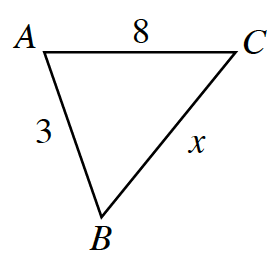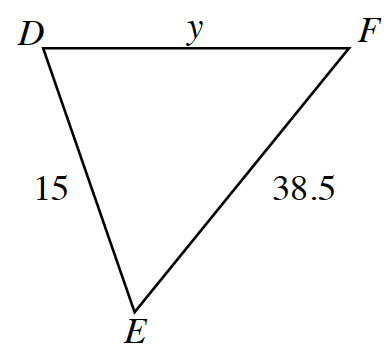### Home > CCAA > Chapter 7 Unit 6 > Lesson CC2: 7.2.2 > Problem7-113

7-113.

Triangle $ABC$ is similar to triangle $DEF$.

1. Find the scale factor from triangle $ABC$ to triangle $DEF$.

Find the scale factor by using two corresponding sides.

The scale factor is $5$.

2. Find $x$.

Divide by the scale factor.

$x=\frac{38.5}{5}$

3. Find $y$.

Multiply the corresponding side by the scale factor.